2802：小游戏

## 2802:小游戏

1000ms

65536kB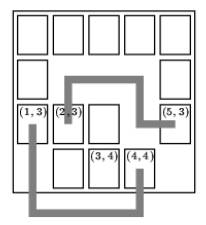``````5 4
XXXXX
X   X
XXX X
XXX
2 3 5 3
1 3 4 4
2 3 3 4
0 0 0 0
0 0
``````

``````Board #1:
Pair 1: 4 segments.
Pair 2: 3 segments.
Pair 3: impossible.

#include <bits/stdc++.h>
using namespace std;
int w,h;
char s;
bool mark;
int direction={{-1,0},{0,1},{1,0},{0,-1}};
int totalStep=100000;
void findTotalPath(int x1,int y1,int x2,int y2,int direct,int step){
//cout<<x1<<" "<<y1<<","<<x2<<" "<<y2<<endl;
if(step>totalStep)
return;
if(x1==x2&&y1==y2){
if(step<totalStep){
totalStep=step;
}
return;
}
for(int i=0;i<4;i++){
/*if((direct==0&&i==2)||(direct==1&&i==3)||(direct==2&&i==0)||(direct==3&&i==1))
continue;*/
int yy1=y1+direction[i];
int xx1=x1+direction[i];
//if((xx1<0)||(yy1<0)||(xx1>=h+2)||(yy1>=w+2)||(mark[yy1][xx1]==true)||((s[yy1][xx1]=='X')&&(yy1!=y2||xx1!=x2))) continue;
/*cout<<x1<<" -"<<y1<<endl;
cout<<xx1<<" "<<yy1<<endl;*/
if((xx1>-1)&&(xx1<w+2)&&(yy1>-1)&&(yy1<h+2)&&(((s[yy1][xx1]==' ')&&(mark[yy1][xx1]==false))||((xx1==x2)&&(yy1==y2)&&(s[yy1][xx1]=='X')))){
//if ((xx1 > -1) && (xx1< w + 2) && (yy1> -1) && (yy1 < h + 2)&& (((s[y][x] == ' ') && (mark[y][x] == false))||((xx1==x2)&& (yy1==y2) && (s[y][x] == ‘X’)))){
mark[yy1][xx1]=true;
if(direct!=i){
findTotalPath(xx1,yy1,x2,y2,i,step+1);
}else{
findTotalPath(xx1,yy1,x2,y2,i,step);
}
mark[yy1][xx1]=false;
}
}
}
int main(){
int id=1;
while(1){
/*cin>>w>>h;
cin.ignore();*/
scanf("%d %d",&w,&h);
if(w==0&&h==0) break;
/*for(int i=0;i<h+2;i++){
s[i]=s[i][w+1]=' ';
}
for(int j=0;j<w+2;j++){
s[j]=s[w+1][j]=' ';
}*/
for (int i = 0; i <100; i ++) s[i] =s[i] = ' ';
for(int i=1;i<h+1;i++){
getchar();
//string str="";
//getline(cin,str);
for(int j=1;j<w+1;j++){
//s[i][j]=str[j-1];
s[i][j]=getchar();
}
}
for (int i = 0; i <= w; i ++)
s[h + 1][i + 1] = ' ';
for (int i = 0; i <= h; i ++)
s[i + 1][w + 1] = ' ';
cout<<"Board #"<<id<<":"<<endl;
id++;
int x1,y1,x2,y2;
int subId=0;
while(1){
subId++;
totalStep=100000;
memset(mark, false, sizeof(mark));
cin>>x1>>y1>>x2>>y2;
if(x1==0&&y1==0&&x2==0&&y2==0) break;
int step=0;
int direct=-1;
findTotalPath(x1,y1,x2,y2,direct,step);
if(totalStep<100000)
cout<<"Pair "<<subId<<": "<<totalStep<<" segments."<<endl;
else{
cout<<"Pair "<<subId<<": "<<"impossible."<<endl;
}
}
cout<<endl;
}
}
``````blmius
1年前
MySQL:[Err] 1292 - Incorrect datetime value: ‘0000-00-00 00:00:00‘ for column ‘CREATE_TIME‘ at row 1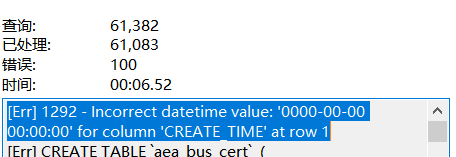Stella981
1年前
ASMSupport教程4.9 生成三元运算符
<p这节我们介绍如何用ASMSupport生成三元运算符(...?...:...)运算符。我们预计生成如下代码：</p<divid"scid:9D7513F9C04C4721824A2B34F0212519:935e30cc33214e0093ba9834f3a4e044"class"wlWriterEditableS天翼云开发者社区
2个月前
SPDK QOS机制解析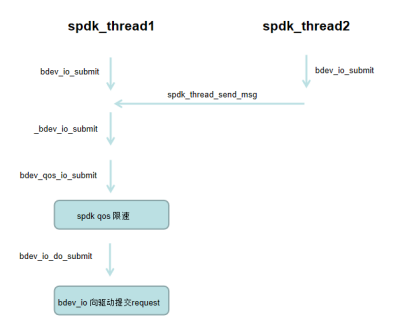天翼云开发者社区
2个月前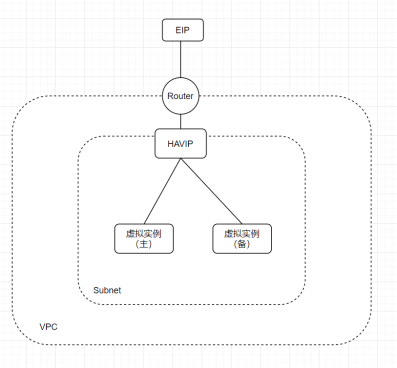天翼云开发者社区
2个月前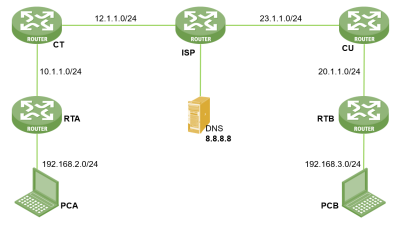天翼云开发者社区
2个月前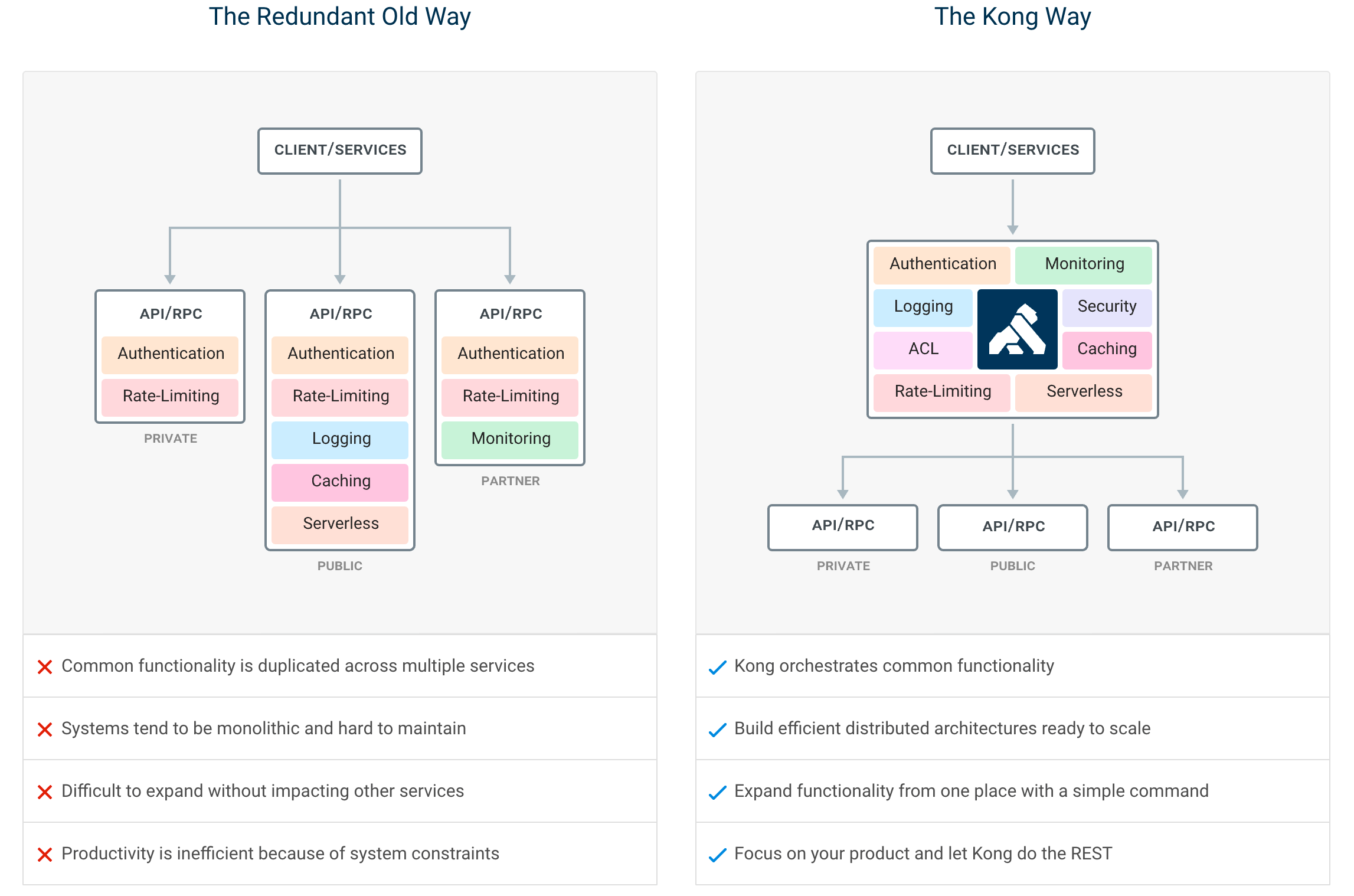天翼云开发者社区
2个月前
SPDK对接Ceph性能优化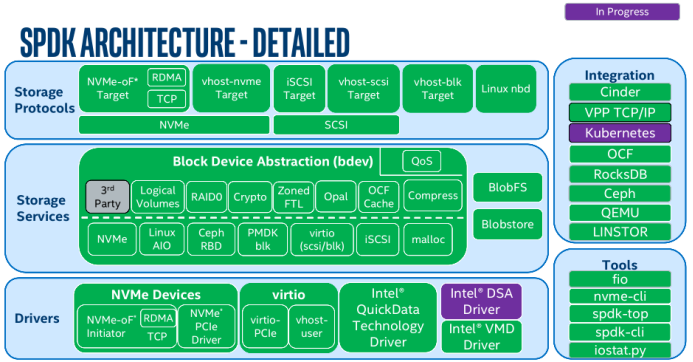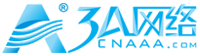3A网络
6个月前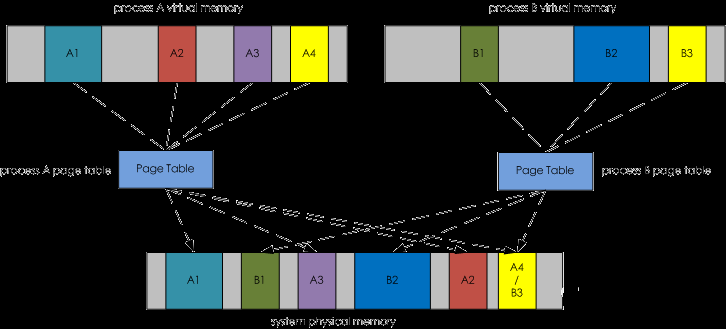3A网络
6个月前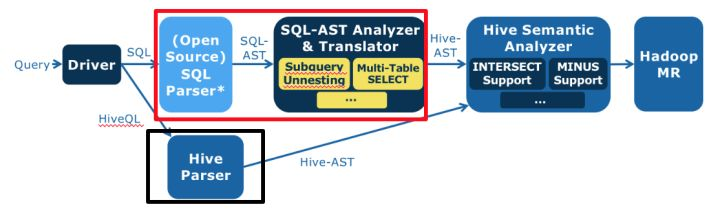天翼云开发者社区
2个月前Wesley13
Lv1

1.2w

12

9